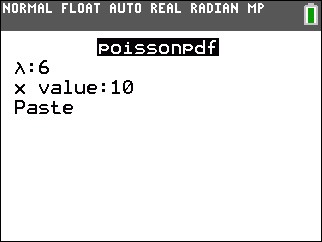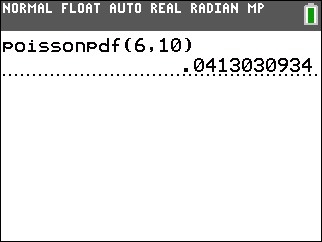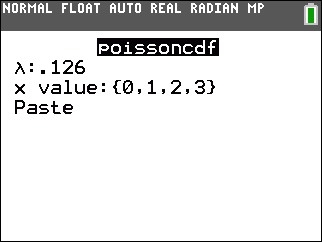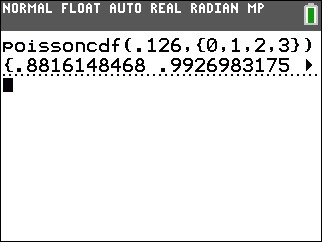# Knowledge Base

## Solution 34600: Computing a Poisson PDF and Poisson CDF on TI-84 Plus C Silver Edition.

### How do I use the poissonpdf( ) or possioncdf( ) command on a TI-84 Plus C Silver Edition?

The command poissoncdf( computes a cumulative probability at x for the discrete Poisson distribution with a specified mean Ã. Please note that Ã must be any real number that is greater than zero; x may be any real number or a list of real numbers. The syntax is as follows:

poissoncdf(Ã, x)

For example, given Ã=6 and x=10 find the probability for the discrete Poisson distribution:

Example 1:

• Press [2nd] [DISTR].
• Press [ALPHA] [C].
• Press  [Enter]   [ENTER].• Highlight Paste and press [Enter].
• Press [ENTER] again.Example 2:

Given Ã=.126 and x={0,1,2,3} find the probability for the discrete Poisson distribution:

• Press [2nd] [DISTR].
• Press [ALPHA][D].
• Press [.]   [ENTER].
• Press [2ND] [{ ]  [,]  [,]  [,] [ }] [ENTER].• Highlight Paste and press [ENTER].
• Press [ENTER] again.Please see the TI-84 Plus C Silver Edition guidebook for additional information.